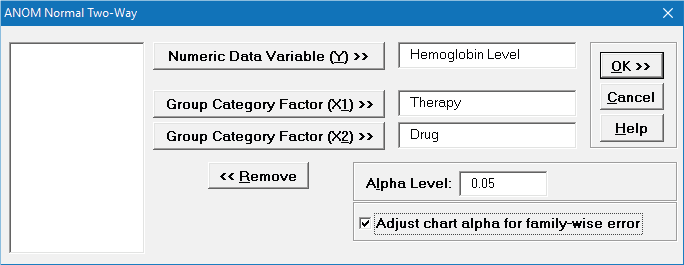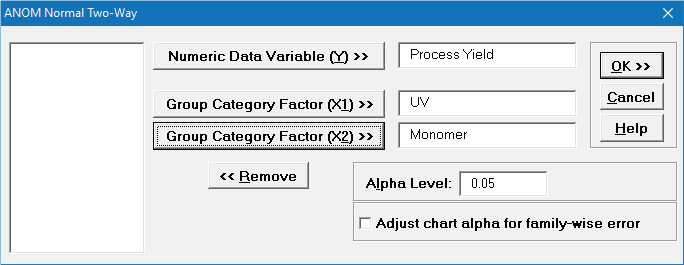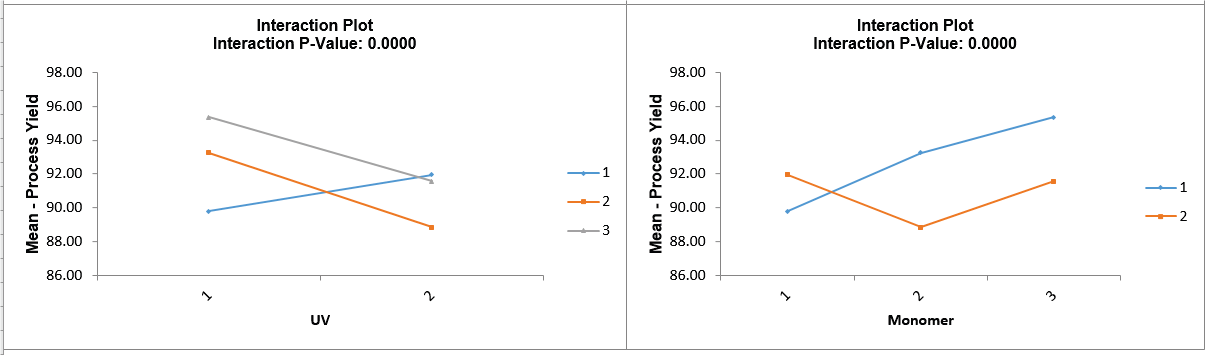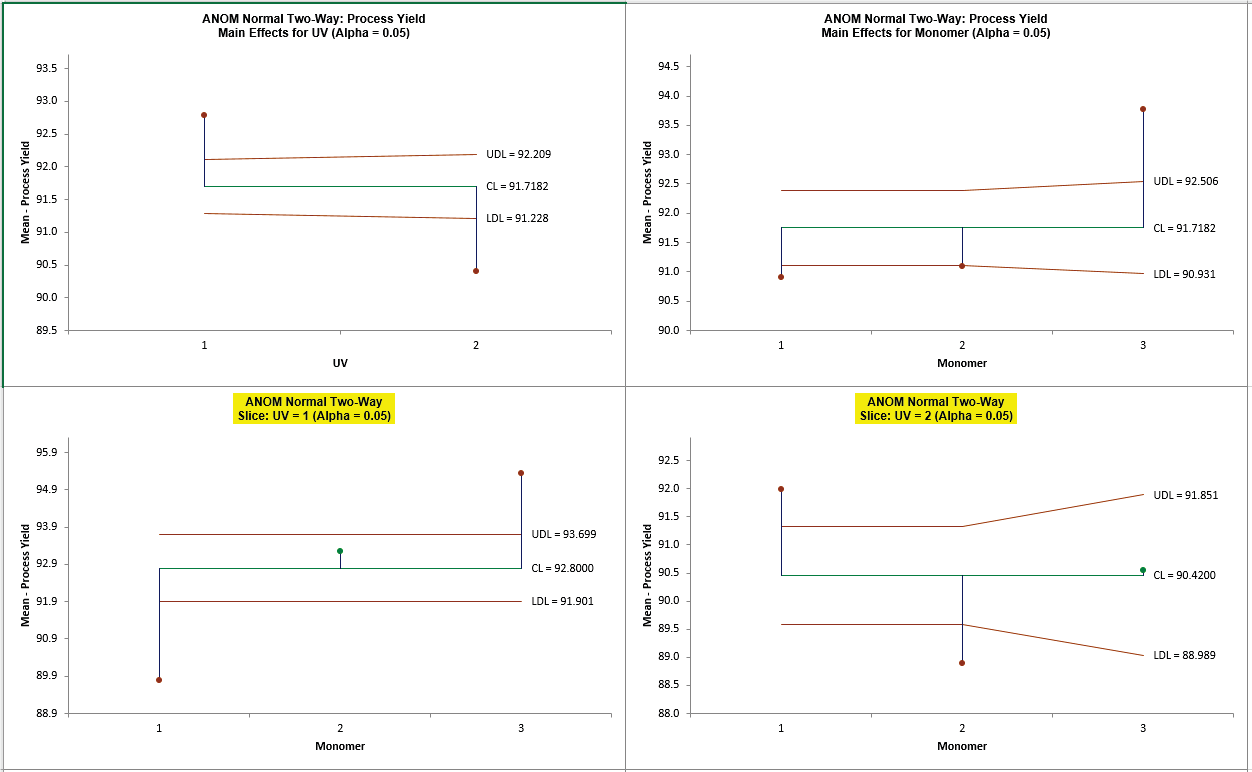Include Top

# How Do I Perform ANOM Normal Two-Way in Excel Using SigmaXL?

1. We will look at two balanced examples from the ANOM book (used with author permission),
one with no interaction and another with a strong interaction, and then a slightly unbalanced
version of the latter (Wludyka, 2013).

2. Open ANOM Examples.xlsx, click on Hemoglobin – Normal tab. This data is from a factorial
design to study hemoglobin levels in males (Example 5.1). The factors are Therapy (Chemo or
Radiation) and Drug (Type 1, 2, 3).
Ensure that the entire data table is selected. If not, check Use Entire Data Table.

3. Click SigmaXL > Graphical Tools > Analysis of Means (ANOM) > ANOM Normal Two-Way.
Ensure that the entire data table is selected. If not, check Use Entire Data Table.

4. Click Next. Select Hemoglobin Level, click Numeric Data Variable (Y) >>; select Therapy, click
Group Category Factor (X1) >>; select Drug, click Group Category Factor (X2) >>. Alpha Level =
0.05
, Adjust chart alpha for family-wise error rate is unchecked:5. Click OK. The ANOM Normal Two-Way Main Effects, Slice charts and Interaction plots are
shown below:6. Since the Interaction is not significant (P-Value is >= 0.1), the Main Effects charts are
highlighted. The decision limits match the manual calculations given in the ANOM book (pages
87, 88). Since the average hemoglobin level for radiation falls below the lower decision limit,
and the average for chemotherapy falls above the upper decision limit, therapy has an (α =
0.05) effect. There is also an effect on hemoglobin level due to the drug since the average
hemoglobin level for drug 3 is above the upper decision line.

Tip: Two-Way ANOM Normal is complimentary to Two-Way ANOVA. Analyzing the above data
using Two-Way ANOVA shows that Therapy is significant with a P-Value = 0.0001, Drug is also
significant with a P-Value = 0.0105 and the Interaction is not significant, with a P-Value =
0.5942.

7. Click Recall SigmaXL Dialog menu or press F3 to recall last dialog. Check Adjust chart alpha for
family-wise error rate.This will apply a Bonferroni correction to the specified alpha values to control the overall
family-wise error rate for the charts. So, the Main Effects alpha will be = 0.05/2 = 0.025 and the
Slice Charts alpha will be = 0.05/2 = 0.025 as well.

8. Click OK. The ANOM Normal Two-Way Main Effects and Slice charts are shown below:9. With the Bonferroni corrected alpha of 0.025, the significant results do not change, but they are
closer to the decision lines. The trade-off for this chart family-wise adjustment is loss of power.

10. In ANOM Examples.xlsx, click on Process Yield – Normal tab. This data is from a factorial
design to study the effect of three monomers and two levels of UV exposure (1 = UV; 2 = No
UV) on the percent yield for contact lens production (Example 5.3).

11. Click SigmaXL > Graphical Tools > Analysis of Means (ANOM) > ANOM Normal Two-Way.
Ensure that the entire data table is selected. If not, check Use Entire Data Table.

12. Click Next. Select Process Yield, click Numeric Data Variable (Y) >>; select UV, click Group
Category Factor (X1) >>
; select Monomer, click Group Category Factor (X2) >>. Alpha Level =
0.05, Adjust chart alpha for family-wise error rate is unchecked:13. Click OK. The ANOM Normal Two-Way Main Effects, Slice charts and Interaction plots are
shown below:14. Since the Interaction P-Value is < 0.1, the Slice charts are highlighted. The decision limits for
the Slice charts match the calculations given in Wludyka (2013). See Appendix References for
Analysis of Means (ANOM) Charts. The Slice plot clearly shows the effect of the strong
interaction. When UV = 1 (UV Exposure), the three monomers differ. In particular, the average
for monomer 1 is below the overall average and the average for monomer 3 is above the
overall average. However, for UV = 2 (no UV), the monomers also differ but in an entirely
different manner than in the UV = 1 case, revealing the nature of the interaction.

Tip: As previously noted, Two-Way ANOM Normal is complimentary to Two-Way ANOVA. Analyzing the above data using Two-Way ANOVA shows that UV is significant with a P-Value = 0.0001, Monomer is significant with a P-Value = 0.0000 (4.4 e-5) and the Interaction is also
significant, with P-Value = 0.0000 (2.2e-6).

15. In ANOM Examples.xlsx, click on Process Yield – Normal Unbal tab. This data is from a factorial design to study the effect of three monomers and two levels of UV exposure (1 = UV; 2
= No UV) on the percent yield for contact lens production, but two of the observations are
missing for A = 2 (no UV) and B = 3 (monomer 3).

16. Click SigmaXL > Graphical Tools > Analysis of Means (ANOM) > ANOM Normal Two-Way.
Ensure that the entire data table is selected. If not, check Use Entire Data Table.

17. Click Next. Select Process Yield, click Numeric Data Variable (Y) >>; select UV, click Group
Category Factor (X1) >>
; select Monomer, click Group Category Factor (X2) >>. Alpha Level =
0.05, Adjust chart alpha for family-wise error rate is unchecked:18. Click OK. The ANOM Normal Two-Way Main Effects, Slice charts and Interaction plots are shown below:19. The significant results of the experiment have not changed, but the decision limits are now
varying due to the unbalanced data and use critical values for unbalanced data. The decision
limits for the Slice charts match the calculations given in Wludyka (2013).

# Web Demos

Our CTO and Co-Founder, John Noguera, regularly hosts free Web Demos featuring SigmaXL and DiscoverSim